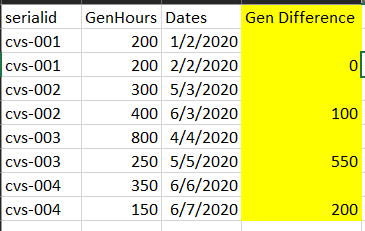cancel
Showing results for
Did you mean:Helper II

## Find difference between the 2 rows of column based on another column criteriaI would want to find the difference in the GenHours for each serialid. Currently this is how my table looks on power BI. I have filtered the latest and 2nd latest date for each serialid.

Now i would want to find the difference between the GenHours that is being diplayed on Power BI after applying filters like removing blanks.

1 ACCEPTED SOLUTIONCommunity Support

According to your statement, I know you want to show absolute Gen Difference. And you will filter your table to show first 2 date for each serialid, you just want show difference in 1st latest date and difference in 2nd latest date will show blank. Here I suggest you build a table visual and create a measure to achieve your goal. Measure could be dynamic.

My Sample:Rank is a calculated column.

``Rank = RANKX(FILTER('Table','Table'[serialid]=EARLIER('Table'[serialid])),'Table'[Dates],,DESC,Dense)``

Build a table visual and filter visual by rank column to show items when value is less than or equal to 2.

Measure:

``````Gen Difference =
VAR _RANK1GenHours =
CALCULATE (
SUM ( 'Table'[GenHours] ),
FILTER (
ALL ( 'Table' ),
'Table'[serialid] = MAX ( 'Table'[serialid] )
&& 'Table'[Rank] = 1
)
)
VAR _RANK2GenHours =
CALCULATE (
SUM ( 'Table'[GenHours] ),
FILTER (
ALL ( 'Table' ),
'Table'[serialid] = MAX ( 'Table'[serialid] )
&& 'Table'[Rank] = 2
)
)
RETURN
IF (
SUM ( 'Table'[Rank] ) = 1,
ABS ( _RANK1GenHours - _RANK2GenHours ),
BLANK ()
)``````

Result is as below.Best Regards,
Rico Zhou

If this post helps, then please consider Accept it as the solution to help the other members find it more quickly.

2 REPLIES 2Community Support

According to your statement, I know you want to show absolute Gen Difference. And you will filter your table to show first 2 date for each serialid, you just want show difference in 1st latest date and difference in 2nd latest date will show blank. Here I suggest you build a table visual and create a measure to achieve your goal. Measure could be dynamic.

My Sample:Rank is a calculated column.

``Rank = RANKX(FILTER('Table','Table'[serialid]=EARLIER('Table'[serialid])),'Table'[Dates],,DESC,Dense)``

Build a table visual and filter visual by rank column to show items when value is less than or equal to 2.

Measure:

``````Gen Difference =
VAR _RANK1GenHours =
CALCULATE (
SUM ( 'Table'[GenHours] ),
FILTER (
ALL ( 'Table' ),
'Table'[serialid] = MAX ( 'Table'[serialid] )
&& 'Table'[Rank] = 1
)
)
VAR _RANK2GenHours =
CALCULATE (
SUM ( 'Table'[GenHours] ),
FILTER (
ALL ( 'Table' ),
'Table'[serialid] = MAX ( 'Table'[serialid] )
&& 'Table'[Rank] = 2
)
)
RETURN
IF (
SUM ( 'Table'[Rank] ) = 1,
ABS ( _RANK1GenHours - _RANK2GenHours ),
BLANK ()
)``````

Result is as below.Best Regards,
Rico Zhou

If this post helps, then please consider Accept it as the solution to help the other members find it more quickly.Super User

@axk180022 See my article on Mean Time Between Failure (MTBF) which uses EARLIER: http://community.powerbi.com/t5/Community-Blog/Mean-Time-Between-Failure-MTBF-and-Power-BI/ba-p/3395....
The basic pattern is:
Column =
VAR __Current = [Value]
VAR __PreviousDate = MAXX(FILTER('Table','Table'[Date] < EARLIER('Table'[Date])),[Date])

VAR __Previous = MAXX(FILTER('Table',[Date]=__PreviousDate),[Value])
RETURN
__Current - __Previous

Become an expert!: Enterprise DNA
External Tools: MSHGQM
Latest book!:
Mastering Power BI 2nd EditionDAX is easy, CALCULATE makes DAX hard...Announcements#### Exclusive opportunity for Women!

Join us for a free, hands-on Microsoft workshop led by women trainers for women where you will learn how to build a Dashboard in a Day!#### Power Platform Conference-Power BI and Fabric Sessions

Join us Oct 1 - 6 in Las Vegas for the Microsoft Power Platform Conference.Top Solution Authors
Top Kudoed Authors
Users online (1,804)